Check Full Chapter Explained - Continuity and Differentiability - Application of Derivatives (AOD) Class 12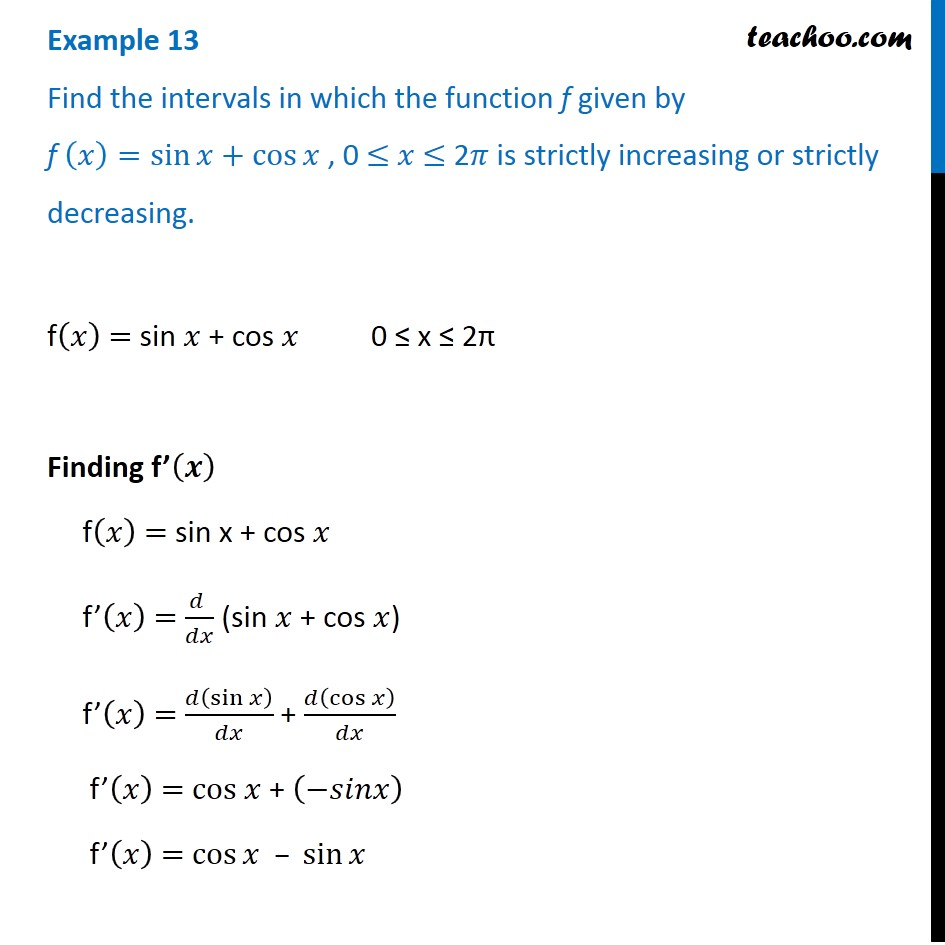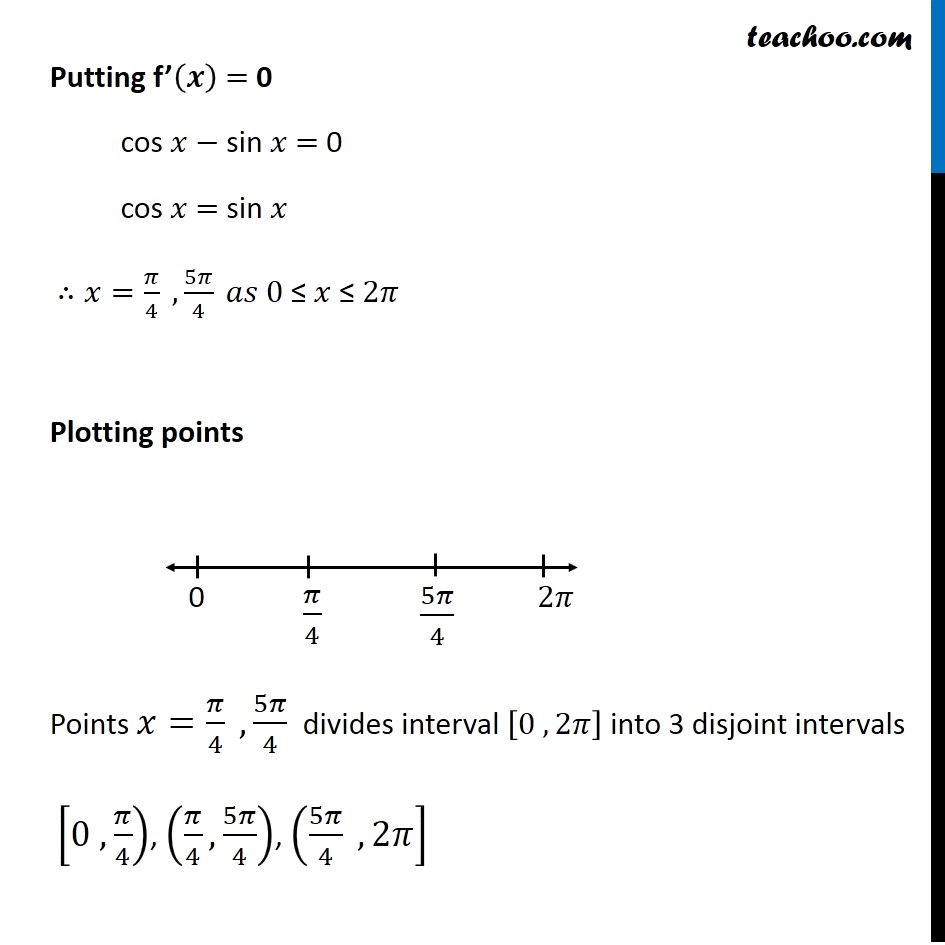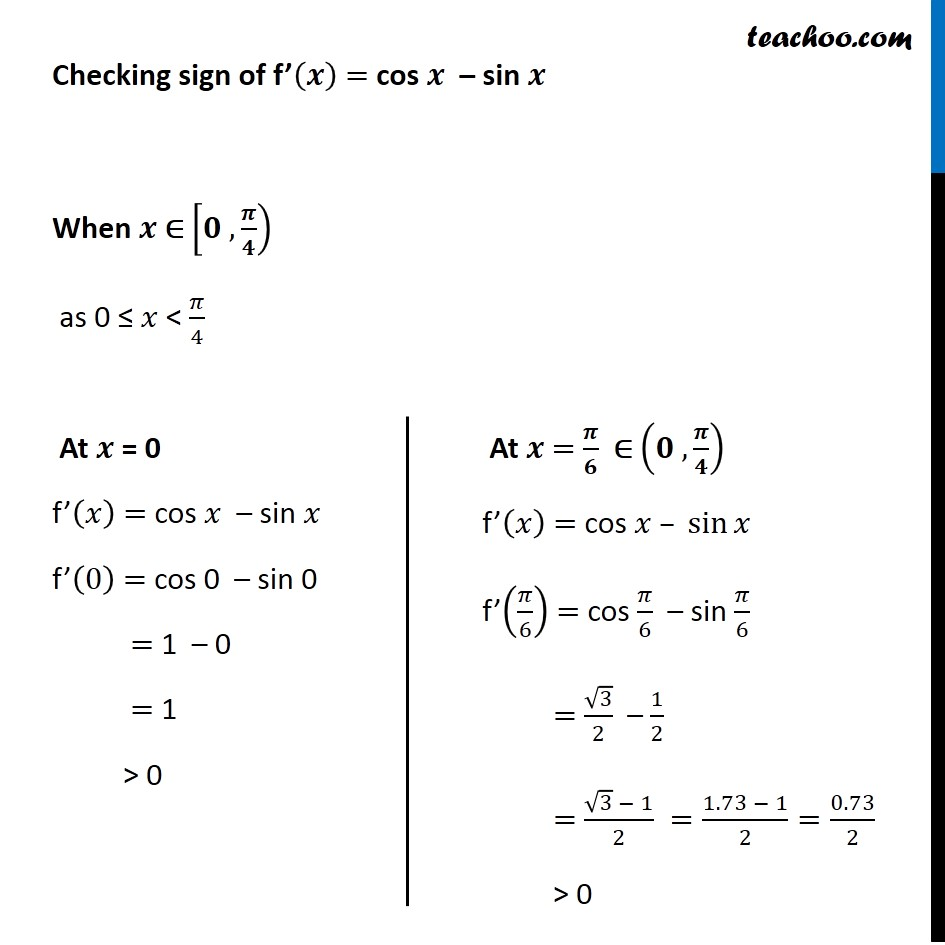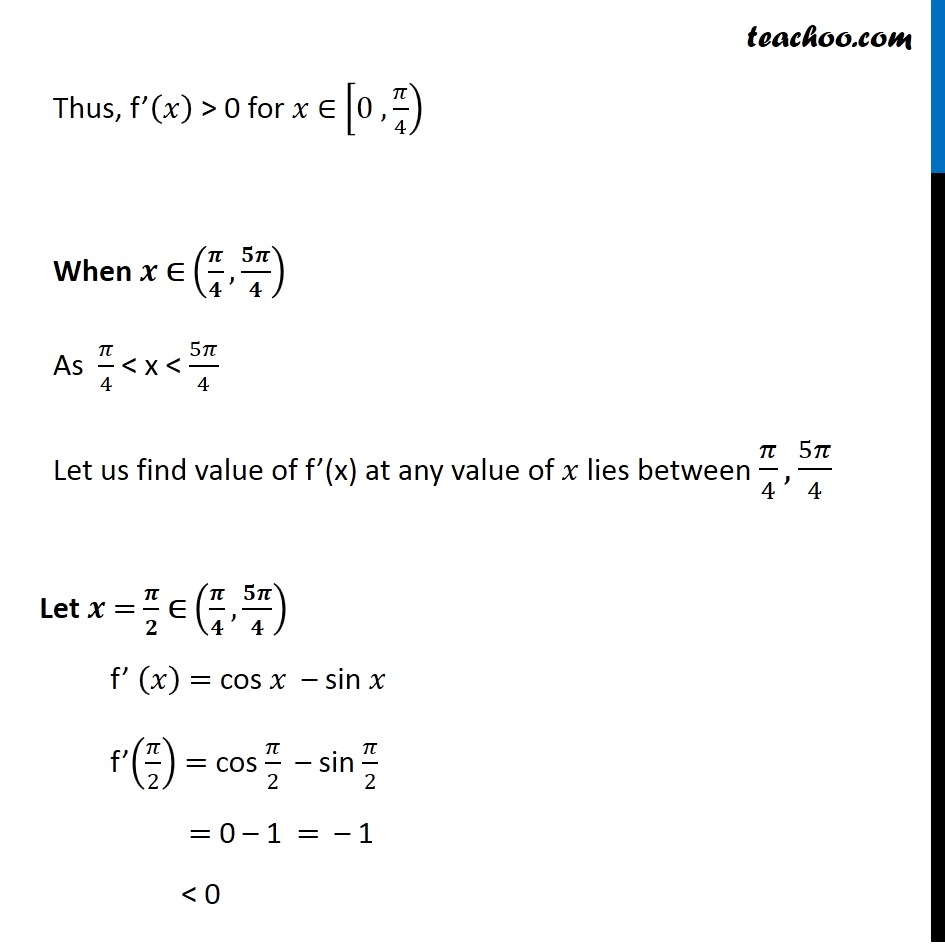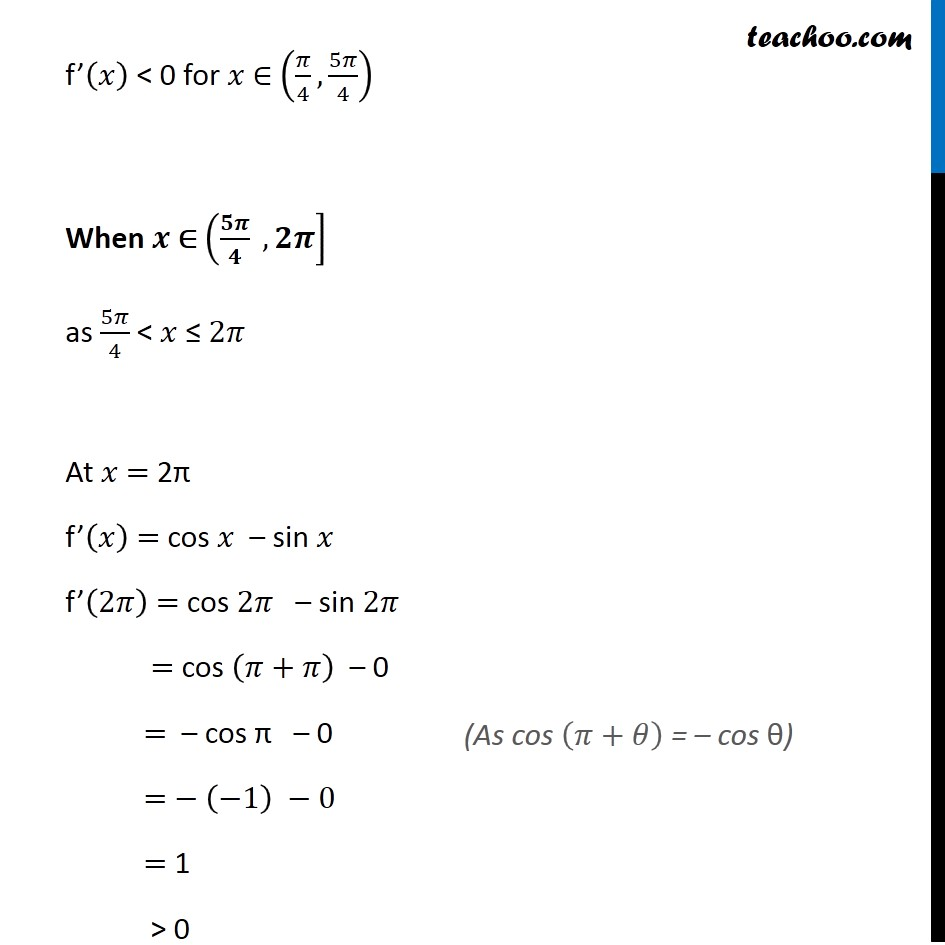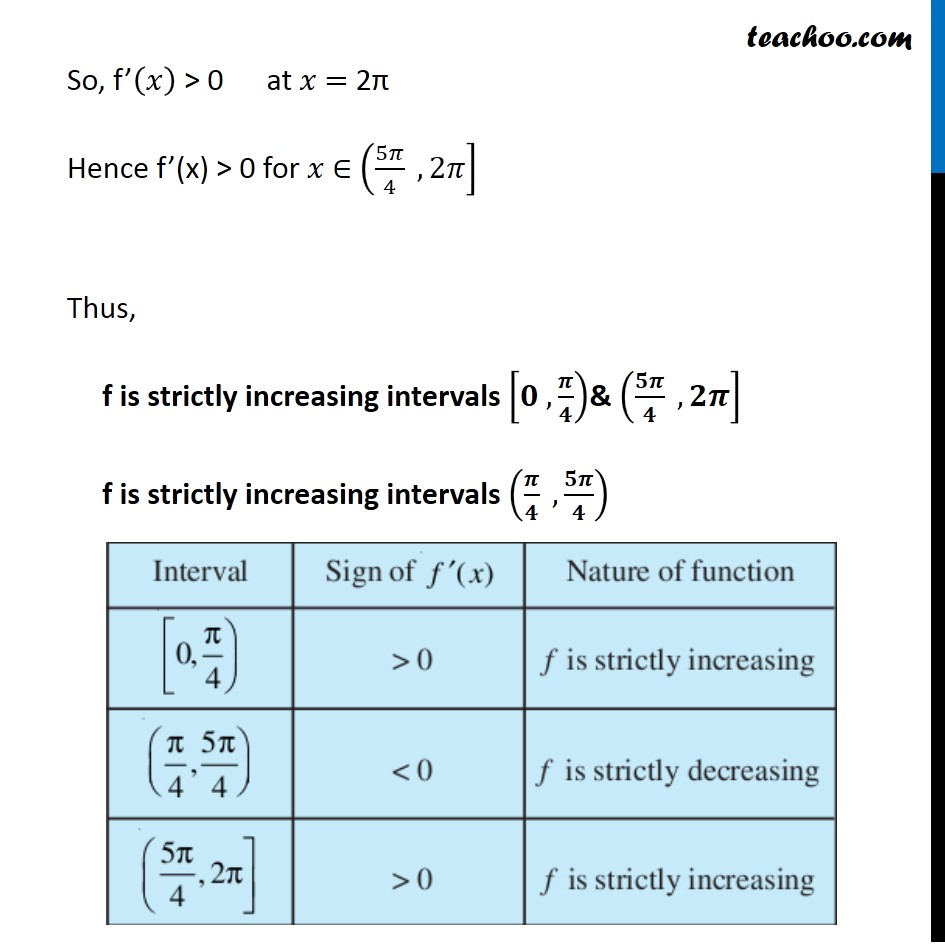1. Chapter 6 Class 12 Application of Derivatives
2. Serial order wise
3. Examples

Transcript

Example 13 Find the intervals in which the function f given by f (𝑥)=sin⁡𝑥+cos⁡𝑥 , 0 ≤ 𝑥 ≤ 2𝜋 is strictly increasing or strictly decreasing. f(𝑥) = sin 𝑥 + cos 𝑥 0 ≤ x ≤ 2π Finding f’(𝒙) f(𝑥) = sin x + cos 𝑥 f’(𝑥) = (𝑑 )/𝑑𝑥 (sin 𝑥 + cos 𝑥) f’(𝑥) = 𝑑(sin⁡𝑥 )/𝑑𝑥 + 𝑑(cos⁡𝑥 )/𝑑𝑥 f’(𝑥) = "cos " 𝑥 + (−𝑠𝑖𝑛𝑥) f’(𝑥) = cos⁡𝑥 – sin⁡𝑥 Putting f’(𝒙) = 0 cos 𝑥 − sin 𝑥 = 0 cos 𝑥 = sin 𝑥 ∴𝑥=𝜋/4 ,5𝜋/4 𝑎𝑠 0" ≤ " 𝑥 ≤ 2𝜋 Plotting points Points 𝑥=𝜋/4 ,5𝜋/4 divides interval [0 , 2𝜋] into 3 disjoint intervals [0 , 𝜋/4), (𝜋/4,5𝜋/4), (5𝜋/4 , 2𝜋] Checking sign of f’(𝒙) = cos 𝒙 – sin 𝒙 When 𝒙 ∈ [𝟎 , 𝝅/𝟒) as 0 ≤ 𝑥 < 𝜋/4 At 𝒙 = 0 f’(𝑥) = cos 𝑥 – sin 𝑥 f’(0) = cos 0 – sin 0 = 1 – 0 = 1 > 0 At 𝒙 = 𝝅/𝟔 ∈ (𝟎 , 𝝅/𝟒) f’(𝑥) = cos 𝑥 – sin⁡𝑥 f’(𝜋/6) = cos 𝜋/6 – sin 𝜋/6 = √3/2 – 1/2 = (√3 − 1)/2 =(1.73 − 1)/2=0.73/2 > 0 Thus, f’(𝑥) > 0 for 𝑥 ∈ [0 , 𝜋/4) When 𝒙 ∈ (𝝅/𝟒,𝟓𝝅/𝟒) As 𝜋/4 < x < 5𝜋/4 Let us find value of f’(x) at any value of 𝑥 lies between 𝜋/4, 5𝜋/4 Let 𝒙 = 𝝅/𝟐 ∈ (𝝅/𝟒,𝟓𝝅/𝟒) f’ (𝑥) = cos 𝑥 – sin 𝑥 f’(𝜋/2) = cos 𝜋/2 – sin 𝜋/2 = 0 – 1 = – 1 < 0 f’(𝑥) < 0 for 𝑥 ∈ (𝜋/4,5𝜋/4) When 𝒙 ∈ (𝟓𝝅/𝟒 , 𝟐𝝅] as 5𝜋/4 < 𝑥 ≤ 2𝜋 At 𝑥 = 2π f’(𝑥) = cos 𝑥 – sin 𝑥 f’(2𝜋) = cos 2𝜋 – sin 2𝜋 = cos (𝜋+𝜋) – 0 = – cos π – 0 = − (−1) −0 = 1 > 0 (As cos (𝜋+𝜃) = – cos θ) So, f’(𝑥) > 0 at 𝑥 = 2π Hence f’(x) > 0 for 𝑥 ∈ (5𝜋/4 , 2𝜋] Thus, f is strictly increasing intervals [𝟎 , 𝝅/𝟒)& (𝟓𝝅/𝟒 , 𝟐𝝅] f is strictly increasing intervals (𝝅/𝟒 , 𝟓𝝅/𝟒)

Examples